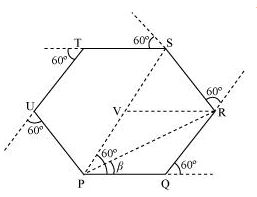## Pages

### Motion in a plane NCERT Solutions Class 11 Physics - Solved Exercise Question 4.10

Question 4.10:

On an open ground, a motorist follows a track that turns to his left by an angle of 60after every 500 m. Starting from a given turn, specify the displacement of the motorist at the third, sixth and eighth turn. Compare the magnitude of the displacement with the total path length covered by the motorist in each case.

Solution:

The path followed by the motorist is a regular hexagon with side 500 m, as shown in the given figureLet the motorist start from point P.
The motorist takes the third turn at S.
∴Magnitude of displacement = PS = PV + VS = 500 + 500 = 1000 m
Total path length = PQ + QR + RS = 500 + 500 +500 = 1500 m
The motorist takes the sixth turn at point P, which is the starting point.
∴Magnitude of displacement = 0
Total path length = PQ + QR + RS + ST + TU + UP
= 500 + 500 + 500 + 500 + 500 + 500 = 3000 m
The motorist takes the eight turn at point R
∴Magnitude of displacement = PR
= ( PQ2 + QR2 + 2 (PQ)(QR) Cos 600)1/2
= ( 5002 + 5002 + 2 X 500 X 500 X Cos 600)1/2
= 866.03 m
β = tan-1 ( 500 Sin 600 / (500 + 500 Cos 600) ) =  300
Therefore, the magnitude of displacement is 866.03 m at an angle of 30° with PR.
Total path length = Circumference of the hexagon + PQ + QR
= 6 × 500 + 500 + 500 = 4000 m
The magnitude of displacement and the total path length corresponding to the required turns is shown in the given table
 Turn Magnitude of displacement (m) Total path length (m) Third 1000 1500 Sixth 0 3000 Eighth 866.03; 30° 4000